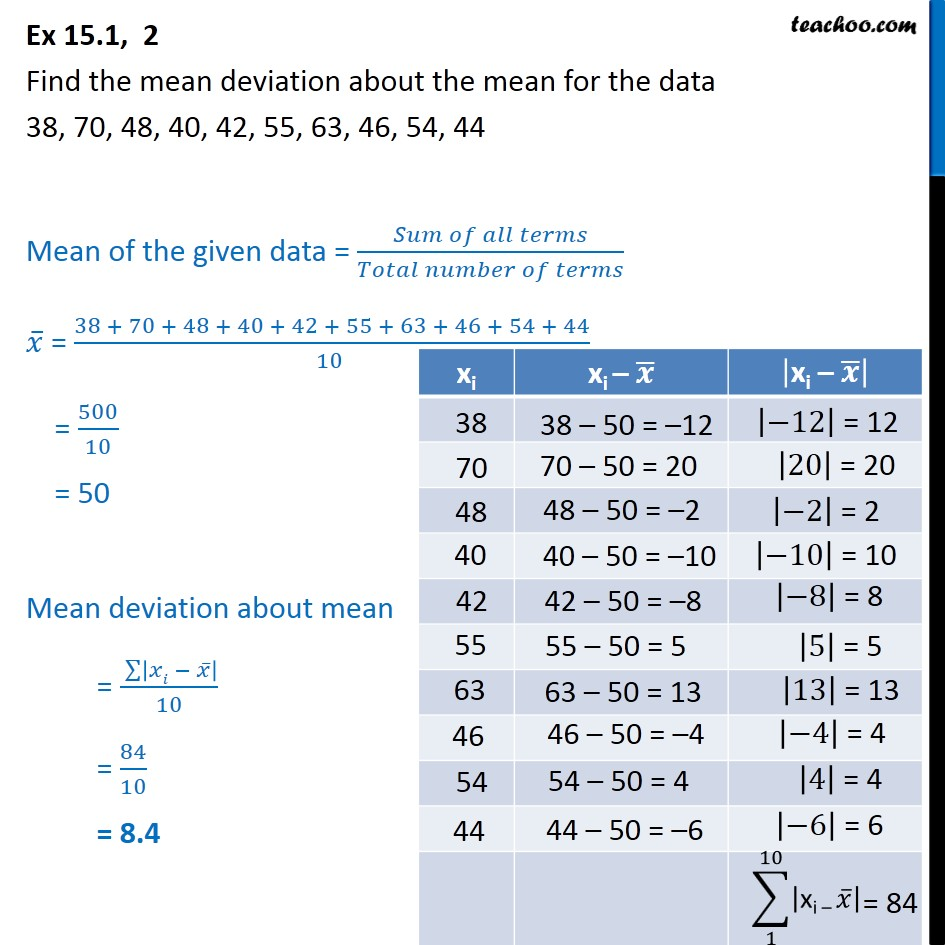Mean deviation about mean - Ungrouped

Chapter 13 Class 11 Statistics
Concept wiseLearn in your speed, with individual attention - Teachoo Maths 1-on-1 Class

### Transcript

Ex 13.1, 2 Find the mean deviation about the mean for the data 38, 70, 48, 40, 42, 55, 63, 46, 54, 44 Mean of the given data = (𝑆𝑢𝑚 𝑜𝑓 𝑎𝑙𝑙 𝑡𝑒𝑟𝑚𝑠)/(𝑇𝑜𝑡𝑎𝑙 𝑛𝑢𝑚𝑏𝑒𝑟 𝑜𝑓 𝑡𝑒𝑟𝑚𝑠) 𝑥 ̅ = (38 + 70 + 48 + 40 + 42 + 55 + 63 + 46 + 54 + 44)/(10 ) = 500/10 = 50 38 38 – 50 = –12 70 70 – 50 = 20 48 48 – 50 = –2 40 40 – 50 = –10 42 42 – 50 = –8 55 63 46 54 44 Mean deviation about mean = ( ∑128▒|𝑥𝑖 − 𝑥 ̅ | )/10 = 84/10 = 8.4